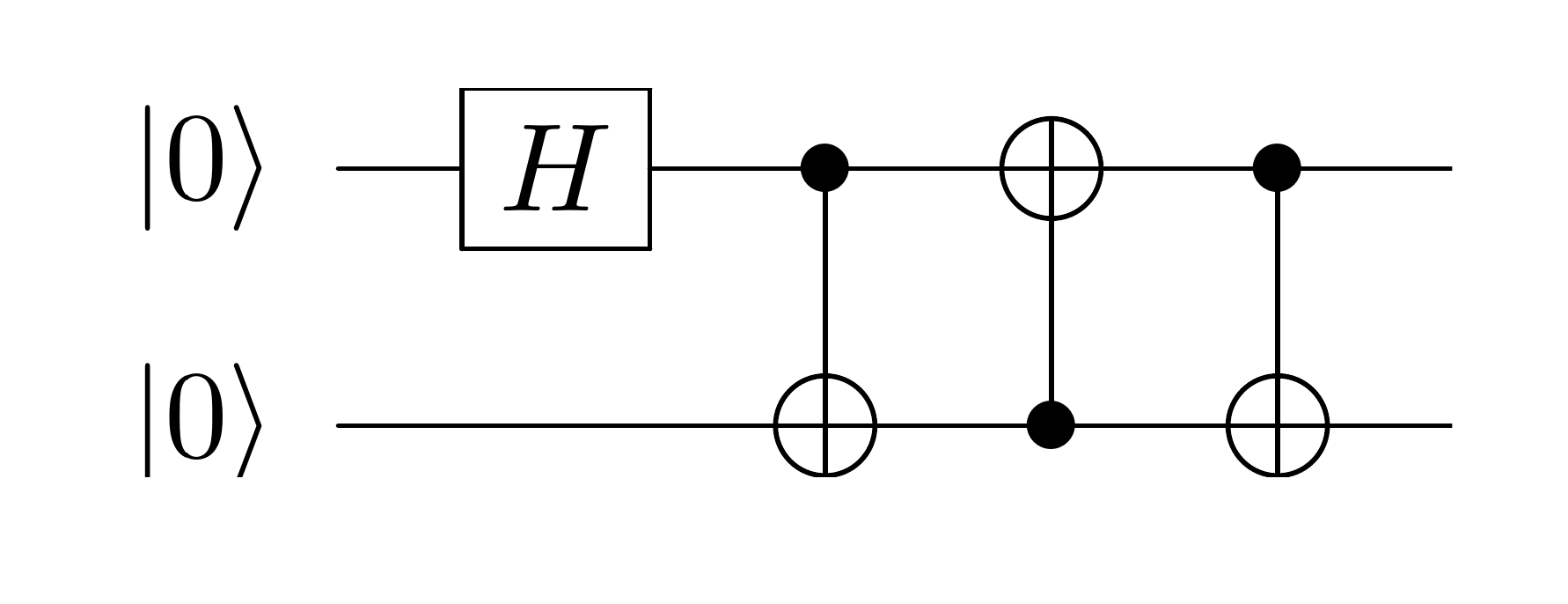# Quantum Homework 3, Thurs 2022-09-08

Due 2022-09-12 4pm in gradescope. Groups of 2 are ok; submit one answer set.

(These questions are from Hidary.)

1. (10 points) Investigate the Stern-Gerlach experiment of 1921. What was the expectation from classical theory for the outcome and what actually occurred?

2. (10 points) What does non-separable mean for two states and what does that tell us about these states?

3. (10 points) For the CZ gate, does it matter which qubit is the control qubit and which is the target?

4. (10 points) What is the final state of the following circuit, in Dirac notation?Total: 40